Chapter 6 Part 1 - Foreign Exchange Rates

Economics Class 12
Macroeconomics

## In Pegging

Exchange Rate of a currency is tied to some other currency or group of currency

Example

Saudi Arabia has pegged its currency Riyal to Dollar since 2003

Peg Rate is 3.75

It means

1 Riyal = 3.75 Dollar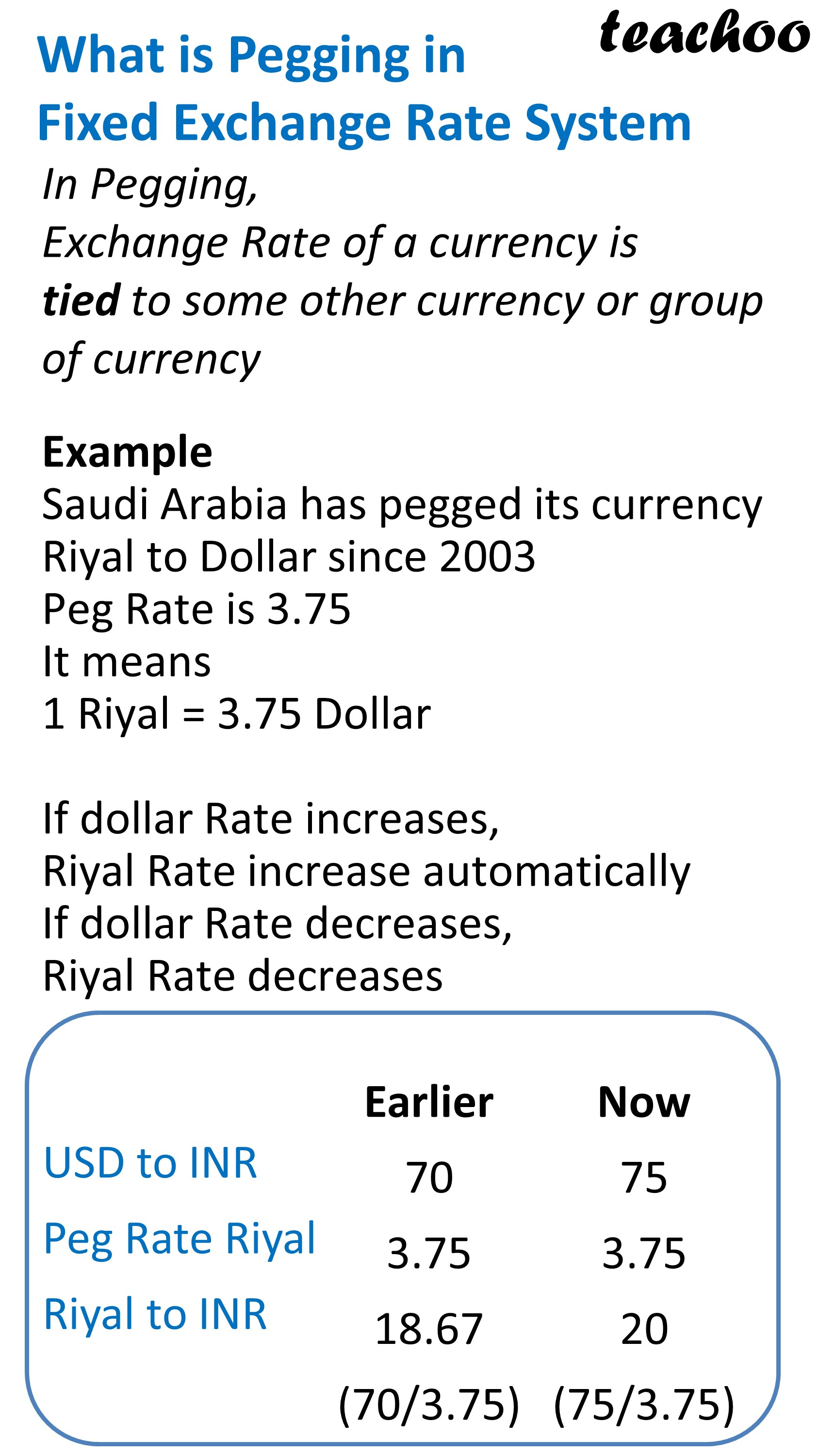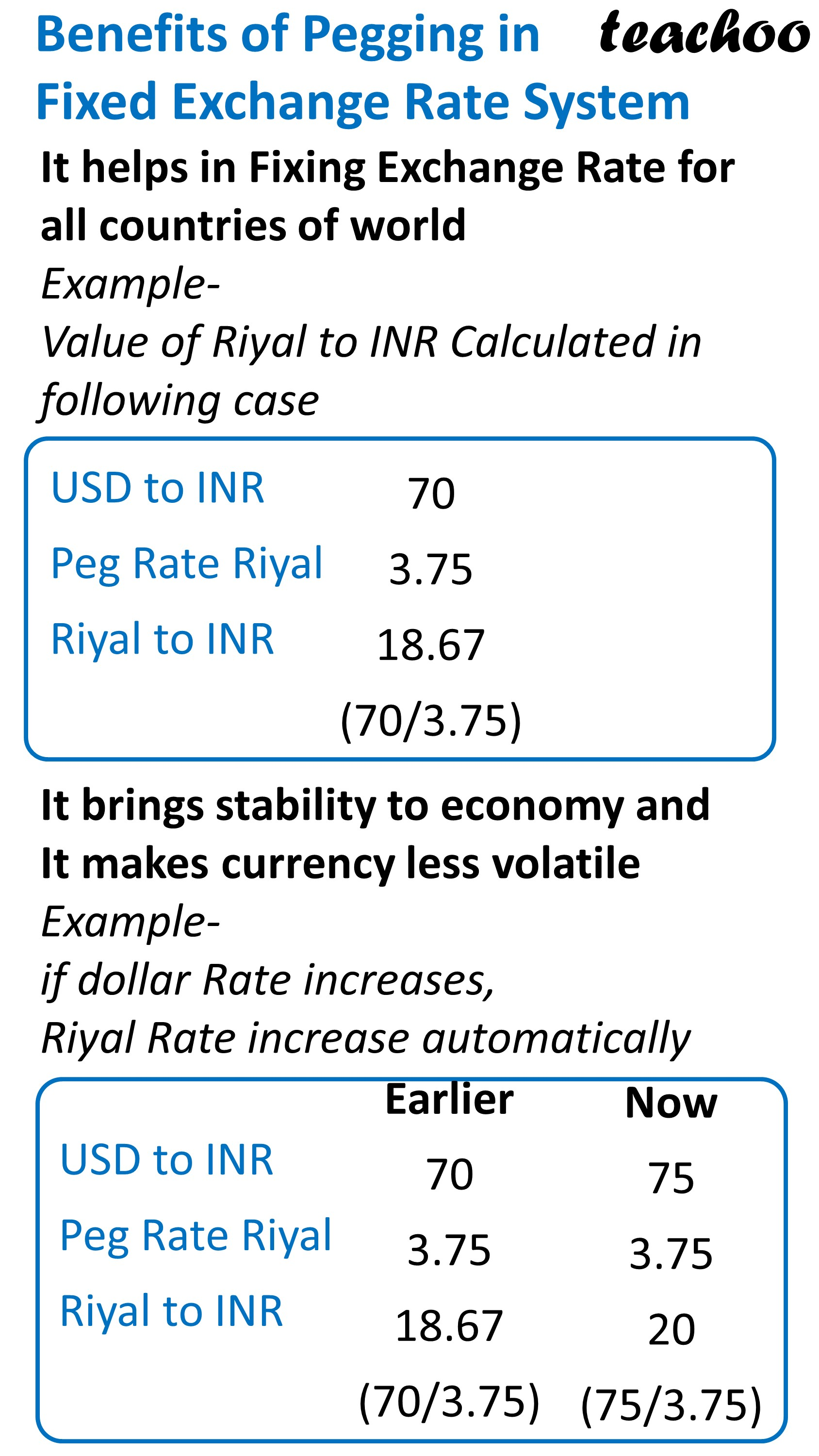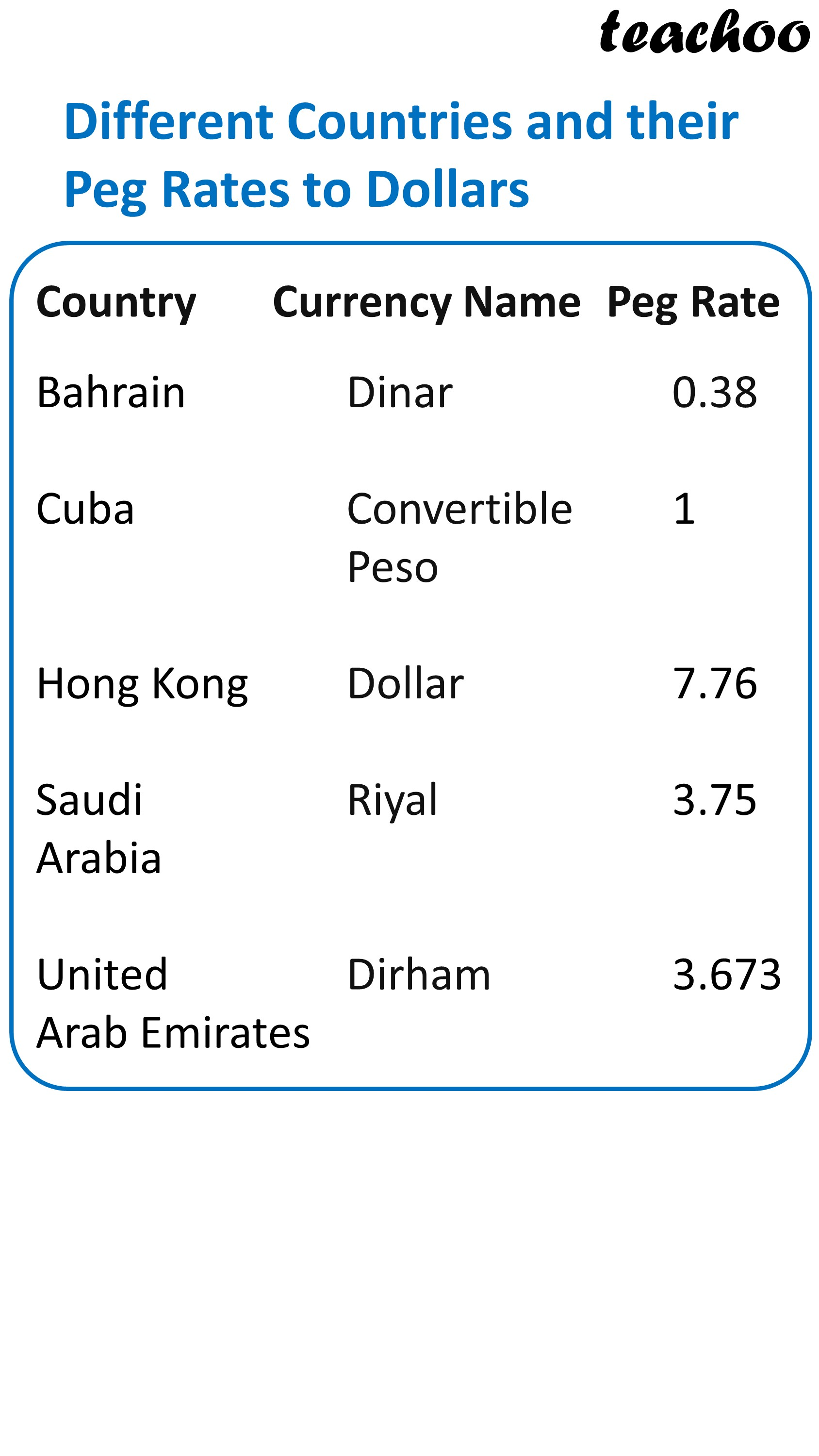### In Parity Value

Exchange Rate of a currency is fixed to an equal value of some commodity

Example

Price of gold in terms of rupee fixed at Rs 4800 /gram

Price of gold in terms of dolar fixed at \$ 60/gram

Hence, Equivalent Price of USA Dollar against rupee= 4800/60 = Rs 80/Dollar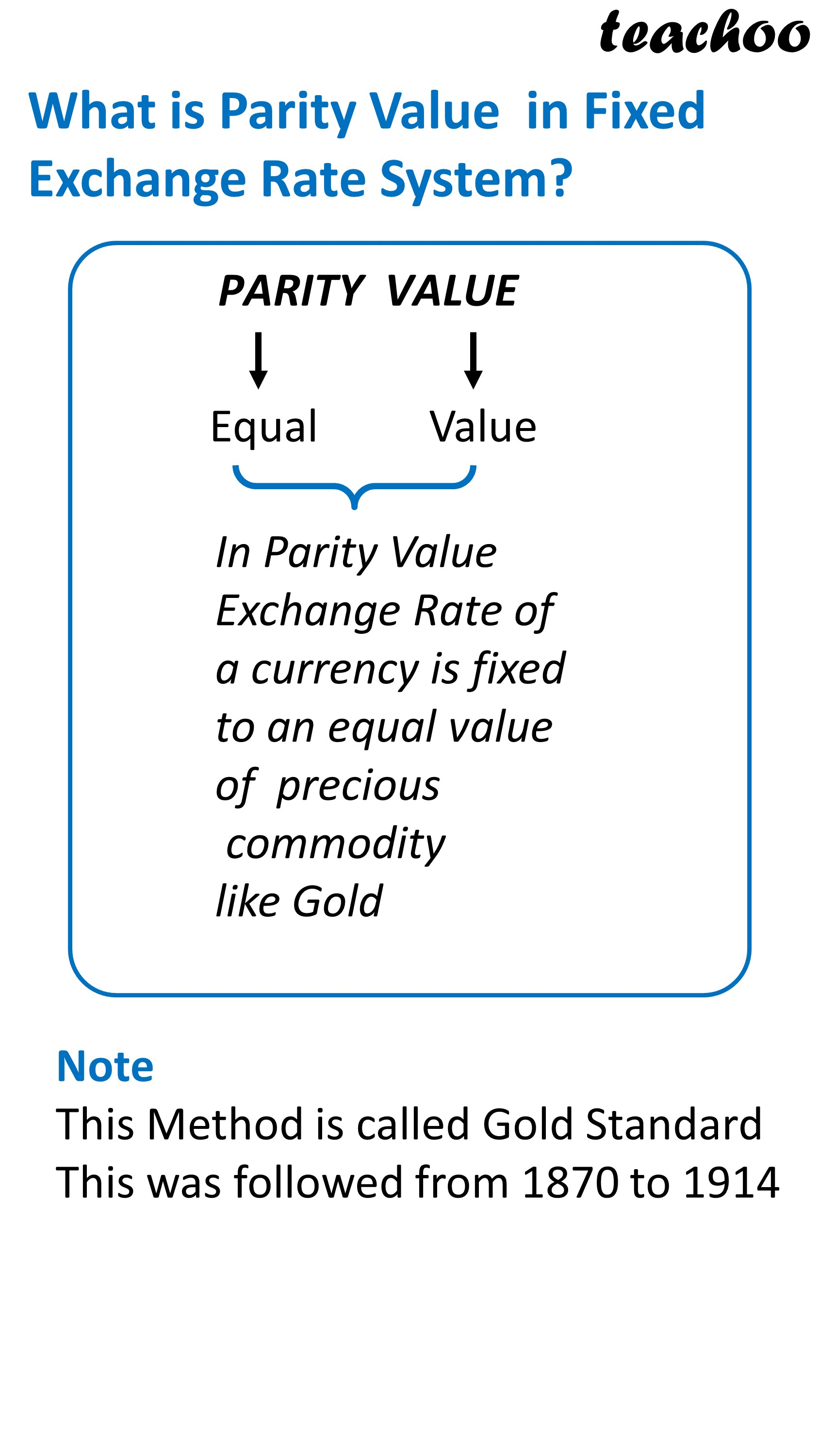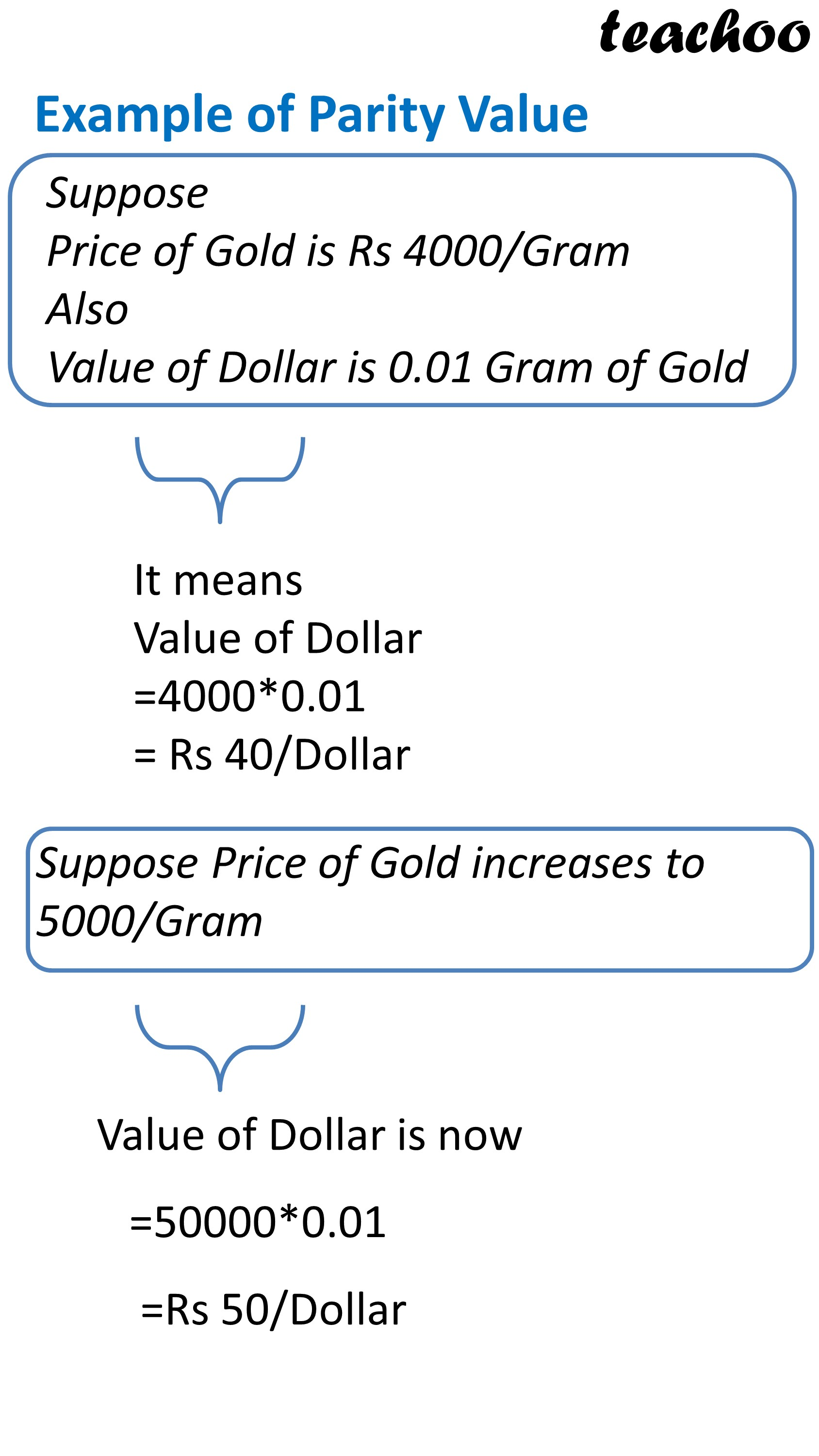Learn in your speed, with individual attention - Teachoo Maths 1-on-1 Class

### Transcript

What is Pegging in Fixed Exchange Rate System In Pegging, Exchange Rate of a currency is tied to some other currency or group of currency Example Saudi Arabia has pegged its currency Riyal to Dollar since 2003 Peg Rate is 3.75 It means 1 Riyal = 3.75 Dollar If dollar Rate increases, Riyal Rate increase automatically If dollar Rate decreases, Riyal Rate decreases USD to INR Peg Rate Riyal Riyal to INR Earlier 70 3.75 18.67 (70/3.75) Now 75 3.75 20 (75/3.75) Benefits of Pegging in Fixed Exchange Rate System It helps in Fixing Exchange Rate for all countries of world Example- Value of Riyal to INR Calculated in following case USD to INR Peg Rate Riyal Riyal to INR 70 3.75 18.67 (70/3.75) It brings stability to economy and It makes currency less volatile Example- if dollar Rate increases, Riyal Rate increase automatically USD to INR Peg Rate Riyal Riyal to INR Earlier 70 3.75 18.67 (70/3.75) Now 75 3.75 20 (75/3.75) Different Countries and their Peg Rates to Dollars Country Bahrain Cuba Hong Kong Saudi Arabia United Arab Emirates Currency Name Dinar Convertible Peso Dollar Riyal Dirham Peg Rate 0.38 1 7.76 3.75 3.673 What is Parity Value in Fixed Exchange Rate System? PARITY VALUE Equal Value In Parity Value Exchange Rate of a currency is fixed to an equal value of precious commodity like Gold Note This Method is called Gold Standard This was followed from 1870 to 1914 Example of Parity Value Suppose Price of Gold is Rs 4000/Gram Also Value of Dollar is 0.01 Gram of Gold It means Value of Dollar =4000*0.01 = Rs 40/Dollar Suppose Price of Gold increases to 5000/Gram Value of Dollar is now =50000*0.01 =Rs 50/Dollar What is Managed Floating Exchange Rate System? Managed (Floating) 2020 2021 2022 Exchange Rate 70.1 70.4 70.3 (Exchange Rate fluctuates frequently but within a Range) It is a mixture of Flexible or Floating Exchange Rate System and Fixed Exchange Rate System Exchange Rate determined by forces of Demand and Supply but Exchange Rate movements are moderated by Central bank Govt It is also called Dirty Floating